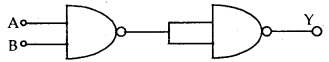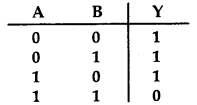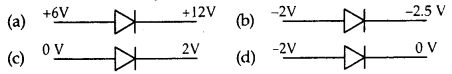# MCQ Questions for Class 12 Physics Chapter 14 Semiconductor Electronics: Materials, Devices and Simple Circuits with Answers

We have compiled the NCERT MCQ Questions for Class 12 Physics Chapter 14 Semiconductor Electronics: Materials, Devices and Simple Circuits with Answers Pdf free download covering the entire syllabus. Practice MCQ Questions for Class 12 Physics with Answers on a daily basis and score well in exams. Refer to the Semiconductor Electronics: Materials, Devices and Simple Circuits Class 12 MCQs Questions with Answers here along with a detailed explanation.

## Semiconductor Electronics: Materials, Devices and Simple Circuits Class 12 MCQs Questions with Answers

Question 1.
In a semiconductor, the forbidden energy gap between the valence band and the conduction band is of the order of:
(a) 1 Mev
(b) 1 ev
(c) 0.1 Mev
(d) 5ev

Question 2.
If the conductivity of a semiconductor is only due to break of the covalent band due to the thermal excitation, then the semiconductor is called:
(a) intrinsic
(b) extrinsic
(c) Acceptor
(d) none of these

Question 3.
In a good conductor, the energy levels in a valence band:
(a) are partially filled only.
(b) overlap with conduction band only.
(c) both (a) and (b) are correct.
(d) none of these

Answer: (c) both (a) and (b) are correct.

Question 4.
A hole in a p-type semiconductor is-
(a) an excess electron
(b) A missing atom
(c) A missing electron
(d) A donor level.

Question 5.
The mobility of conduction electrons is greater than that of holes since electrons is greater than that of holes since electrons.
(a) are negatively charged.
(b) are lighter
(c) require smaller energy for moving through the crystal lattice.
(d) Undergo smaller number of collisions.

Answer: (c) require smaller energy for moving through the crystal lattice.

Question 6.
The Voltage gain is highest for
(a) common emitter amplifier
(b) common base amplifier
(c) common collector amplifier.
(d) Equal in all the three.

Question 7.
In an n-p-n transistor circuit the collector current is 18 mA. If 90% of the electrons emitted reach the collector, than the emitter current is:
(a) 1.6 mA
(b) 16.4 mA
(c) 18 mA
(d) 20 mA

Question 8.
In the common emitter amplifier, the phase difference between the input voltage and output voltage signal across the collector and emitter is:
(a) 0
(b) $$\frac {π}{2}$$
(c) π
(d) $$\frac {π}{4}$$

Question 9.
In common base amplifier, the phase difference between the input and output voltage signal is
(a) 0
(b) $$\frac {π}{2}$$
(c) $$\frac {π}{4}$$
(d) π

Question 10.
The part of the transistor which is heavily doped to produce a large number of majority carriers is:
(a) emitter
(b) base
(c) collector
(d) none

Question 11.
In principle, Boolean algerbra is based on:
(a) simple numbers
(b) binary numbers
(c) logic
(d) truth

Question 12.
The following logic symbol is equivalent to:(a) AND gate
(b) OR gate
(c) NOT gate
(d) NAND gate

Question 13.
Which of the following gates corresponds to the truth table given here:(a) NAND
(b) OR
(c) NOR
(d) AND

Question 14.
The conductivity of semiconductors like Ge and Si:
(a) increases when it is doped with pentavalent impurity.
(b) increases when it is doped with trivalent impurity.
(c) increases when it is doped with pentavalent or trivalent impurity.
(d) none

Answer: (c) increases when it is doped with pentavalent or trivalent impurity.

Question 15.
In which case is the junction diode forward biased.Question 16.
Assuming that the junction diode is ideal, the current in the arrangement shown here is:(a) 2 mA
(b) 30 mA
(c) 20 mA
(d) 10 mA

Question 17.
An oscillator is an amplifier with:
(a) a large gain
(b) Negative feedback
(c) positive feedback
(d) no feedback

Question 18.
How many AND gates are required to form, NAND gate?
(a) 0
(b) 1
(c) 2
(d) 4

Question 19.
Which of following statements is not true?
(a) Resistance of an intrinsic semiconductor decreases with increase in temperature.
(b) Doping pure Si with trivalent impurities gives p-type semiconductor.
(c) The majority carriers in n-type semiconductor are holes.
(d) A p-n junction can act as semiconductor diode.

Answer: (c) The majority carriers in n-type semiconductor are holes.

Question 20.
For a transistor, current amplification factor is 0.8. The transistor is changed to common emitter configuration. For a change of 6 mA in base current. Change in collector current is
(a) 4.8 mA
(b) 6 mA
(b) 8 mA
(d) 24 mA

Question 21.
A truth table is given below. Which of the following has this type of truth table?(a) XOR gate
(b) NOR gate
(b) AND gate
(d) OR gate

Fill in the Blanks

Question 1.
…………………. is called as junction in p-n junction diode.

Answer: The surface of contact of p-type and n-type crystal.

Question 2.
The thickness of the depletion layer is of the order of ………………….

Answer: 1 µm = 10-6 m.

Question 3.
The potential barrier for S1 is about …………………. and the electric field across the junction is about ………………….

Question 4.
The width of the depletion layer and the potential barrier across the junction …………………. due to reverse biasing.

Question 5.
The direction of conventional current in a p-n junction is from …………………. when it is forward biased.

Question 6.
The zener voltage can have value from …………………. to …………………. volts.

Question 7.
The arrow in the symbol of a transistor shows the direction ………………….

Answer: Conventional current or hole current.

Question 8.
Below knee voltage, the variation of current in the p-n junction is …………………. and above it, it is ………………….

Question 9.
Various components such as resistors, inductors, capacitors, transistors, diodes and logic-gates etc. are grown over one ………………….

Question 10.
…………………. is the most developing area where semiconductors are used.

Question 11.
1 and 0 are called ………………….

Question 12.
The connection between logic and mathematics was realised by …………………. and the algebra developed by him based on 0 and 1 is called ………………….

Question 13.
When a p-n junction is forward biased, then the motion of charge carriers across the barrier is due to …………………. and when it is reversed biased, then the motion of charge carriers is due to ………………….

Question 14.
Digital circuits can be obtained by repetitive use of …………………. gates and are called digital building blocks.

Question 15.
In a digital circuit, the diodes and transistors are operated by a 5V supply. The states 1 and 0 will correspond to …………………. V and …………………. V respectively.

Question 16.
In insulator, the valence elctrons form a band that is ………………….

Answer: valence band which is filled completely.

Question 17.
In the band structure of an intrinsic semiconductor is located ………………….

Answer: midway between the valence band and the conduction band.

Question 18.
The region near the junction of pn—diode where there are no charge carriers is called ………………….

Question 19.
A small impurity is added to Ge to get a p-type semiconductor and this impurity is called ………………….

Question 20.
The Boolean expression for AND gate is ………………….